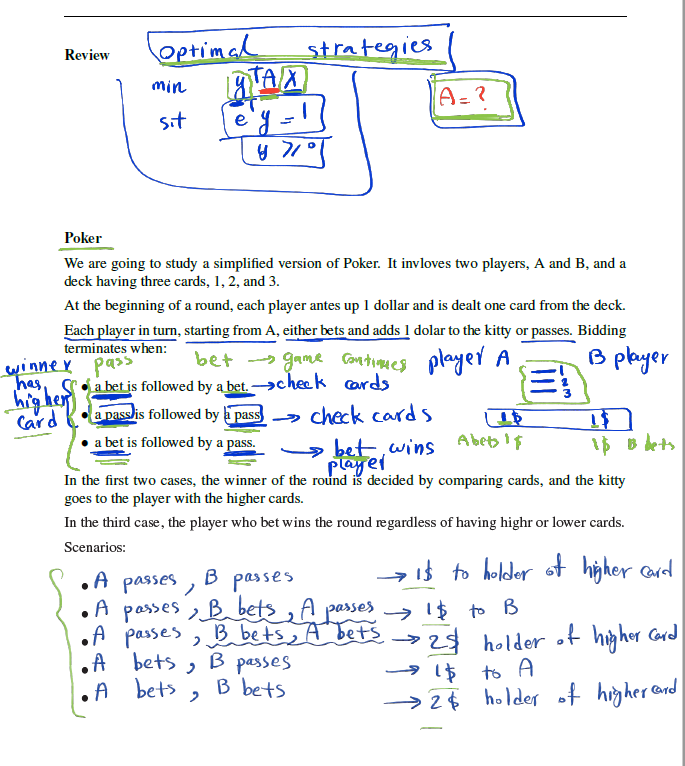## [Math 308] Week 12

midterma가 (3,1,2) 전략을 쓴다는 것은 card1일때 3번 전략을 쓰고, card2일때 1번 전략을 쓰고, card 3일 때 2번 전략을 쓴다는 뜻이다. holiday

2021년 4월 13일
·
0개의 댓글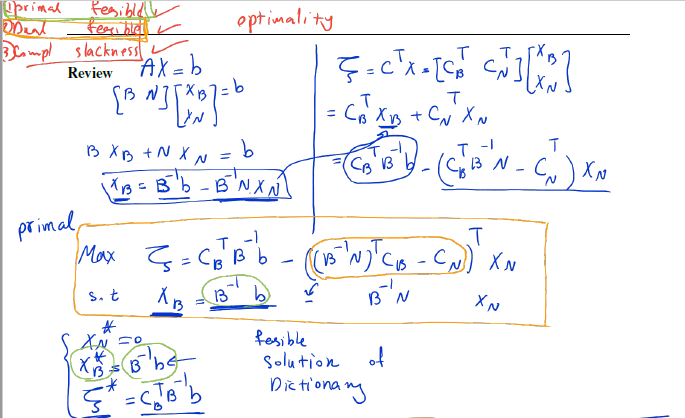## [Math 308] Week 8

primaldualz_n\*가 모두 >=0 이면 dual feasible이라는 것이고, 또 동시에 optimal 하다는 뜻이다. 따라서 1. primal feasible 2. Complementary slackness는 매번 확인되지만 3번은 확인 되는 순간 end o

2021년 3월 17일
·
0개의 댓글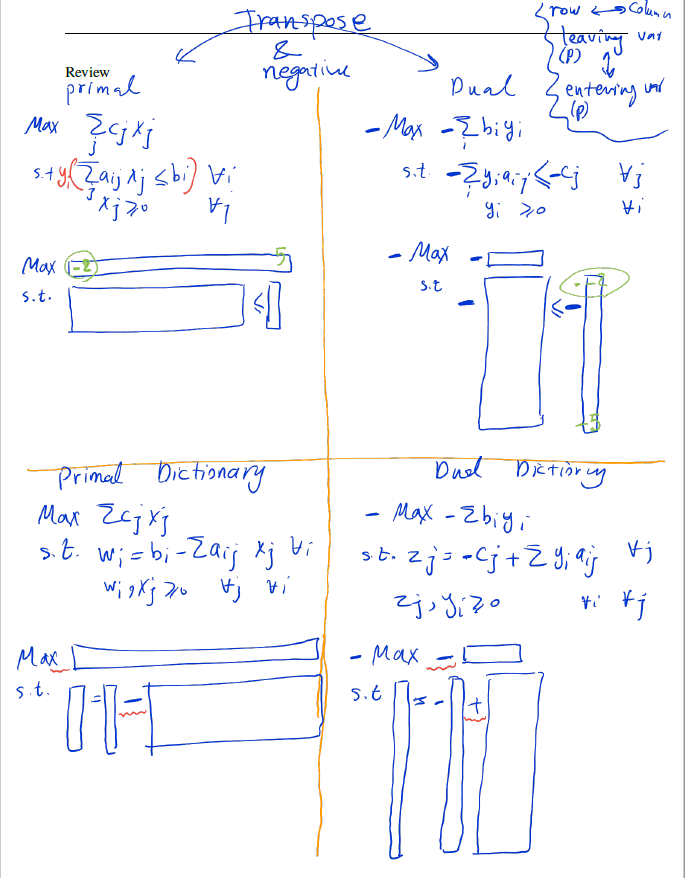## [Math 308] Week 5

Midterm1 As we saw in out discussion of the strong duality theorem, one can actually apply the simplex methodto the dual problem without every writing

2021년 2월 22일
·
0개의 댓글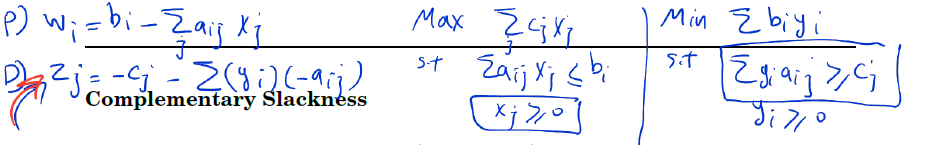## [Math 308] Week 4

Suppose that x = (x_1, x_2, ..., x_n) is primal feasible and that y = (y_1, y_2, ..., y_m) is dual feasible. Let (w_1, w_2, ..., w_m) denote the corre

2021년 2월 12일
·
0개의 댓글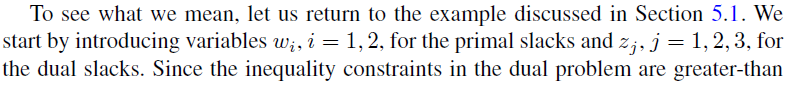## [Math 308] Week 3

Day 1 Performance Measures > For now, we shall measure the amount of effort to solve a linear programming problem by counting the number of iterations

2021년 1월 31일
·
0개의 댓글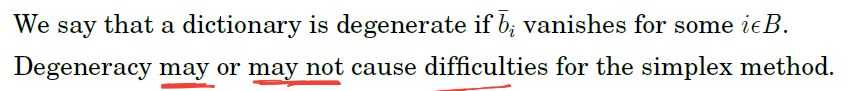## [Math 308] Week 2

In the examples above the degeneracy is not cause any problem. However, problem arises when a degenerate dictionary produces degenerate pivots.feasibl

2021년 1월 24일
·
0개의 댓글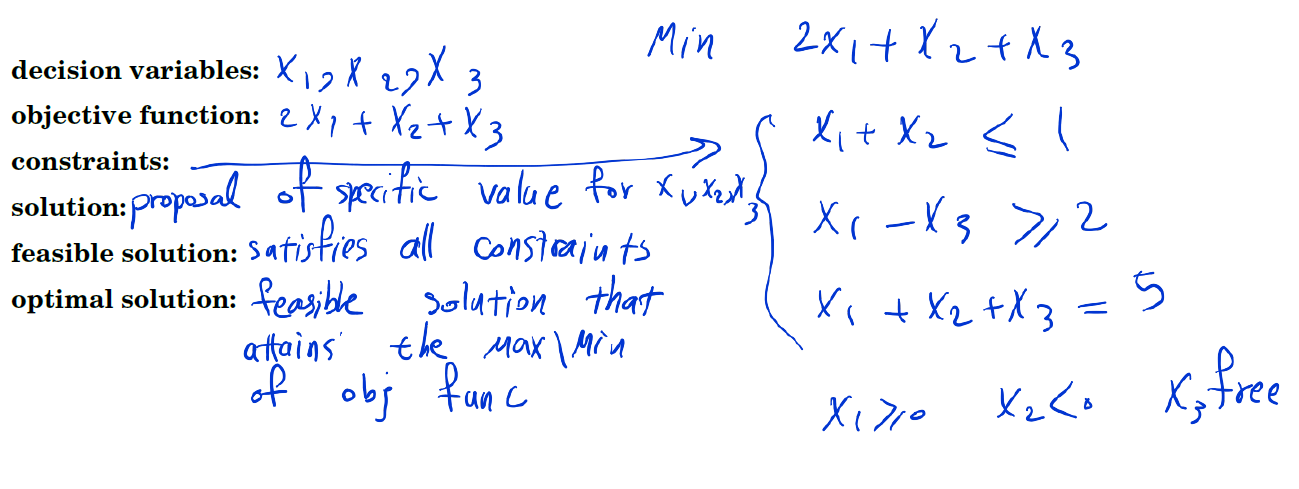## [Math 308] Week 1

Linear programming is concerned with the optimization (minimization or maximization) of a linear function while satisfying a set of linear equality an

2021년 1월 20일
·
0개의 댓글